Name:    Chapter 3 Post Test

Multiple Choice
Identify the choice that best completes the statement or answers the question.

1.

Solve the linear equation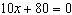for x.
 a.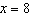b.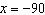c.d.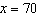e.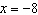2.

The perimeter of a rectangle is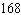inches. The length of the rectangle is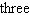times its width. Find the dimensions of the rectangle.
 a. length: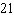incheswidth: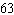inches b. length: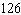incheswidth: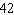inches c. length:incheswidth:inches d. length:incheswidth:inches e. length:incheswidth:inches

3.

Solve the linear equation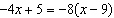for x.
 a.b.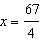c.d.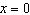e.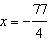4.

Use cross-multiplication to solve the equation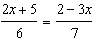for x.
 a.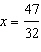b.c.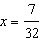d.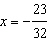e.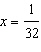5.

Solve the equation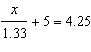for x. Round your answer to two decimal places.
 a.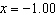b.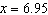c.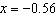d.e.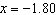6.

Solve the equationfor x. Round your answer to two decimal places.
 a.b.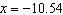c.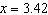d.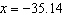e.7.

Convert 33.5% to a fraction.
 a.b.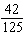c.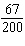d.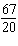e.8.

Convert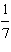% to a fraction.
 a.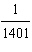b.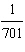c.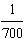d.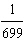e.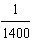9.

Write the ratio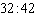as a fraction in simplest form.
 a.b.c.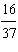d.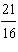e.10.

Find a ratio that compares the relative sizes of the quantities.

11 inches to 8 feet
 a.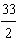b.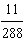c.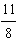d.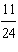e.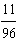11.

Find the unit price (in dollars per ounce) if a 21-ounce can of pineapple costs \$3.36.
 a. \$0.21 per ounce b. \$0.23 per ounce c. \$0.16 per ounce d. \$0.18 per ounce e. \$0.19 per ounce

12.

Solve the proportion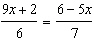to determine x.
 a.b.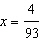c.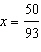d.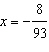e.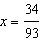13.

If an object traveled 230 miles at a rate of 25 miles per hour, how long (in hours) did it take to travel this distance?
 a. 9.7 hours b. 0.11 hour c. 5750 hours d. 9.2 hours e. 7.7 hours

14.

Suppose \$12,000 is borrowed for 5 years and is to be paid back with interest in one lump sum. If the annual interest rate is 8%, what will the lump sum payment be?
 a. \$16,800.00 b. \$492,000.00 c. \$16,100.00 d. \$17,400.00 e. \$4,800.00

15.

Suppose \$7000 is divided between two investments earning 9% and 5% simple interest. Over the course of a year the two accounts earned a total of \$554. How much was invested in each account?
 a. 1900 in the account earning 9%5100 in the account earning 5% b. 4600 in the account earning 9%2400 in the account earning 5% c. 5100 in the account earning 9%1900 in the account earning 5% d. 3500 in the account earning 9%3500 in the account earning 5% e. 2400 in the account earning 9%4600 in the account earning 5%

16.

Two cars start at the same point and travel in the same direction at average speeds of 60 miles per hour and 40 miles per hour. How much time (in hours) must elapse before the two cars are 60 miles apart?
 a. 3.00 hours b. 0.60 hour c. 1.00 hour d. 1.50 hours e. 2.50 hours

17.

Which inequality has the graph that is shown?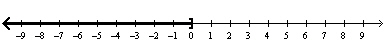a.b.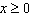c.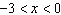d.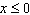e.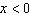18.

Which inequality has the graph that is shown?a.b.c.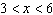d.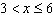e.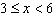19.

Solve the inequality.

 a.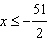b.c.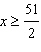d.e.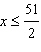20.

A cell phone company offers two text messaging plans. With the first plan, you can send an unlimited number of text messages for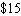a month. With the second plan, you can sendtext messages for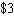and then pay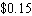for every additional message sent. How many text messages must be sent in a month for the first plan to be less expensive than the second plan?
 a.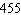b.c.d.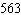e.